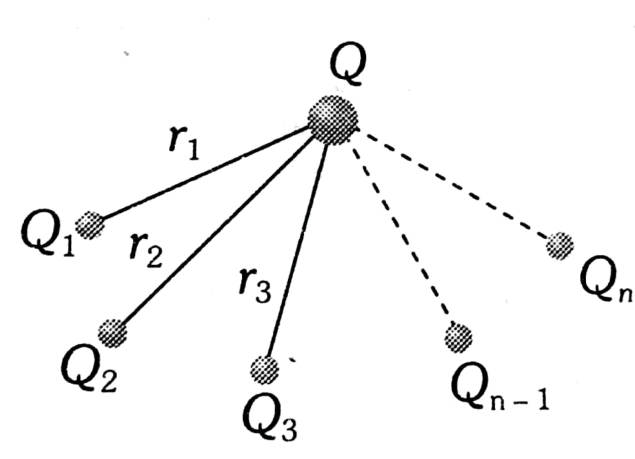#### A charge Q is placed at each of the opposite corners of a square. A charge q is placed at each of the other two corners. If the net electrical force on Q is zero, then the Q/q equals Option 1)Option 2)Option 3)Option 4)As we learnt in

Principle of Super Position -

It states that total force acting on a given charge due to number of charges is the Vector sum of the individual force acting on that charge due to all the charges.

- whereintotal force of attraction along diagonal (takingcomponent)Option 1)This option is correct.

Option 2)This option is incorrect.

Option 3)This option is incorrect.

Option 4)This option is incorrect.

#### prateek